# The Method of Characteristics with applications to Conservation Laws*

Dr. Scott A. Sarra , October 17, 2002

Method of Characteristics Applet

audience: Undergraduate students in a partial differential equations class, undergraduate (or graduate) students in mathematics or other sciences desiring a brief and graphical introduction to the solutions of nonlinear hyperbolic conservation laws or to the method of characteristics for first order hyperbolic partial differential equations.

abstract: This web article and accompanying applet introduces the method of characteristics for solving first order partial differential equations (PDEs). First, the method of characteristics is used to solve first order linear PDEs. Next, the method of characteristics is applied to a first order nonlinear problem, an example of a conservation law. It is discussed why the method may breakdown for nonlinear problems. Difficulties that appear in the nonlinear case, but are absent in the linear case, are examined and the mathematical resolutions of these problems are introduced. Concepts dealing with the solutions of nonlinear hyperbolic conservation laws are introduced and explored graphically. However, the detailed explanation of such concepts may take up entire text books, and the concepts are not explored in detail or with rigor in this short paper. References to more detailed material is given.

* This article and applet appear in the Journal of Online Mathematics and its Applications (JOMA), Volume 3, February 2003.

1 - The Method of Characteristics
1.1 - General Strategy
2 - The Method of Characteristics, special case b(x,t)=1 and c(x,t)=0
2.1 - Constant Coefficient Advection Equation
2.2 - Variable Coefficient Advection Equation
3 - Conservation Laws
3.1 - Inviscid Burgers' Equation
3.2 - Numerical Methods for Conservation Laws
3.3 - Inviscid Burgers' equation example problems
3.3.1 - Riemann problem - shock
3.3.2 - Riemann problem - rarefaction
3.3.3 - Sine
3.3.4 - N wave
3.3.5 - Single hump
4 - Applet
5 - References

## The Method of Characteristics

The method of characteristics is a method which can be used to solve the initial value problem (IVP) for general first order (only contain first order partial derivatives) PDEs. Consider the first order linear PDE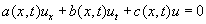(1)

in two variables along with the initial condition. The goal of the method of characteristics, when applied to this equation, is to change coordinates from (x,t) to a new coordinate system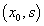in which the PDE becomes an ordinary differential equation (ODE) along certain curves in the x-t plane. Such curves,, along which the solution of the PDE reduces to an ODE, are called the characteristic curves or just the characteristics. The new variable s will vary, and the new variable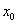will be constant along the characteristics. The variablewill change along the initial curve in the x-t plane (along the line t=0). How do we find the characteristic curves? Notice that if we choose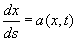(2a) and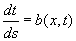(2b) then we have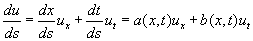and along the characteristic curves, the PDE becomes the ODE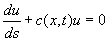. (3)

Equations (2a) and (2b) will be referred to as the characteristic equations.

### General Strategy

The general strategy for applying the method of characteristics to a PDE of the form (1) is: (step 1) Solve the two characteristic equations, (2a) and (2b). Find the constants of integration by setting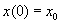(these will be points along the t=0 axis in the x-t plane) and t(0)=0. We now have the transformation fromto,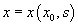and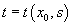. (step 2) Solve the ODE (3) with initial condition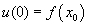, whereare the initial points on the characteristic curves along the t=0 axis in the x-t plane. (step 3) We now have a solution. Solve for s andin terms of x and t (using the results of step 1) and substitute these values into get the solution to the original PDE as.

## The Method of Characteristics, special case b(x,t)=1 and c(x,t)=0

We will examine the method of characteristics for three different PDEs: the constant coefficient advection equation, the variable coefficient advection equation, and inviscid Burgers' equation. For all three examples, the initial conditions are specified as. The examples take a slightly simpler form than the general equation (1). All three examples have b(x,t)=1 and c(x,t)=0. In this case characteristic equation (2b) becomes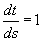. The solution is t=s + k. Using the initial condition t(0)=0, we determine that the constant is k=0, and we have that s=t. In this special case with b(x,t)=1, we only have one characteristic equation to solve. Before proceeding to the examples, let us restate the general strategy in terms of this special case that we are considering in the examples.

(step1) Solve the characteristic equation (2a),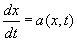, with the initial condition. (step 2) Solve the ODE (3), which in this case simplifies to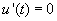, with initial condition(step 3) We now have a solution. Solve forin terms of x and t (using the results of step 1) and substitute forinto get the solution to the original PDE as.

Our first example, the advection equation, is the PDE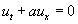(4) where a is a real constant. The constant a is referred to as the wave speed or velocity of propagation. This is the rate at which the solution will propagate along the characteristics. The velocity is constant, so all points on the solution profile will move at the same speed a.

Now we apply the method of characteristics outlined in the 3 steps in the previous section. (step 1) The characteristic equation (2a) to solve iswith the initial condition. The solution gives the characteristic curves,whereis the point where each curve intersects the x-axis in the x-t plane. (step 2) Solve equation (3),with initial condition. The solution is. (step 3). The characteristic curves are determined by,, so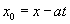, and the solution of the PDE is.

To verify thatdoes indeed remain constant along the characteristics, we differentiatealong one of these curves to find the rate of change of u along the characteristic:So we find that the rate of change is zero, verifying that u is constant along the curve.

By writing the characteristic curves as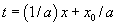it is easy to see that in the x-t plane, the characteristics are parallel lines with slope 1/a. The slope of the characteristics depends only on the constant a. Experiment in the applet with different values (a is changed through the dialog box which is displayed when the parameters button is pressed) of the wave speed, a. Set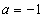to observe the solution profile moving in the opposite direction and observe the negative slope of the characteristics.

The variable coefficient advection equation is(5) where for this example we have taken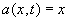. The wave speed depends on the spatial coordinate, x. Thus, the speed of a point on the solution profile will depend on the horizontal coordinate, x, of the point.

(step 1) The characteristic equation (2a) to solve is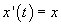with the initial condition. Solving it gives the characteristic curves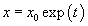. (step 2) Solve equation (3),with initial condition. The solution is. (step 3) The characteristic curves are determined by, so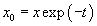and the solution of the PDE in the original variables is.

View the variable coefficient advection equation simulation in the applet. Notice that the characteristics are not straight lines. Also observe that the characteristics do not intersect.

## Conservation Laws

A very important class of partial differential equations is that of conservations laws. As their name indicates, they include those equations that model the conservation laws of physics: mass, momentum, energy, etc. A scalar conservation law in one space dimension takes the form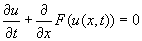(6)

where F(u) is a flux function. In general, conservation laws are nonlinear. For a derivation of the conservation law (6), the interested reader may consult [2, p. 31] or [3 p. 75]. We will use the method of characteristics to examine a one dimensional scalar conservation law, inviscid Burgers' equation, which takes the form of a nonlinear first order PDE. Inviscid Burgers' equation will have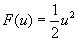.

### Inviscid Burgers' Equation

The inviscid Burgers' equation isor equivalently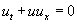. The wave speed depends on the solution,. Thus, the speed of a point on the solution profile will depend on the vertical coordinate, u, of the point. Inviscid Burgers' equation is not of the form of the linear first order PDE (1), as it is nonlinear, so our earlier analysis do not apply directly. However, from our experience with the constant (4) and variable coefficient (5) advection equations, we are led to set the characteristic equation to be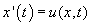. If x(t) is a solution of this equation, then u[x(t),t] is the restriction of u to this curve. Also, along this curve,Thus, this solution u[x(t),t] will not change with time along the curve, so it is a characteristic curve. If we know the initial condition, we can find the characteristic curve by substituting this value into the characteristic equation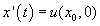. The right hand side of the equation is constant, indicating that the characteristic curves will be straight lines, as in the constant coefficient advection equation case. The characteristic curve will be. The solution of the initial value problem can be written as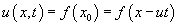. The solution is given implicitly and in all but the simplest cases, it will be impossible to determine the solution explicitly. The characteristics are straight lines, but the lines do not all have the same slope. It will be possible for the characteristics to intersect. If we write the characteristics as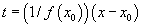, we see that in the x-t plane that they are lines with slope. The slope of the characteristics depends on the pointand on the initial data.

Unlike the first two examples, it is possible for the characteristics to intersect. If the initial data is smooth, then the method of characteristics can be used to determine the solution for small enough t such that the characteristics do not intersect. For larger t, after characteristics have interested, the PDE will fail to have a classical solution (a classical solution is a single valued solution or a function), as the information obtained by following the characteristics will produce a multivalued solution, or possibly, no solution at all. To overcome this lack of existence of a classical solution, we must introduce a broader notion of a solution, a weak solution. Roughly speaking, a weak solution may contain discontinuities, may not be differentiable, and will require less smoothness to be considered a solution than a classical solution. Working with the weak solution of a PDE usually requires that the PDE be reformulated in an integral form. If a classical solution to the problem exists, it will also satisfy the definition of a weak solution.

If the characteristics on both sides of a discontinuity of a piecewise continuous weak solution impinge on the discontinuity curve in the direction of increasing t, the weak solution is called a shock. For inviscid Burgers' equation, the time at which the characteristics cross and a shock forms, the "breaking" time, can be determined exactly as,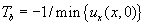[1, p. 25]. The formula can be used if the equation has smooth initial data (so that it is differentiable). From the formula for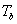, we can see that the solution will break and a shock will form ifis negative at some point. The discontinuous weak solution, in this case a shock wave, will travel at a speed given by the Rankine-Hugoniot condition. (see [1, p. 31] and [2, p. 46]). For inviscid Burgers' equation the shock speed, s, is given by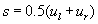where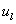is the value of u on the left side of a discontinuity and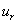is the value of u on the right side of the discontinuity. In addition to characteristics crossing and a shock forming, another possible way the method of characteristics can break down, and a discontinuity can form, is if the characteristics on both sides of the discontinuity emanate from it, rather than go into it. Then the discontinuity is called a rarefaction wave. (see applet simulation and rarefaction example below)

By expanding our class of solutions to include weak solutions, we no longer have uniqueness of the solution of the initial value problem. Additional criteria must be used to pick out the physically correct weak solution. The selection criteria for physically meaningful discontinuous weak solutions is called an entropy condition. A different method to pick out the physically correct weak solution, but one that picks of the same physically correct weak solution as the entropy condition does, is a vanishing viscosity approach. For inviscid Burgers' equation, this would amount to finding solutions to Burgers' equation,, in the limit as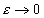. A graphical technique for constructing weak solutions for problems with shocks is the equal area rule ([4, p. 42] and [1, p. 34]). The application of the equal area rule starts with the multivalued solution constructed by using the method of characteristics and then eliminates the multivalued parts by inserting shocks in a way that eliminates the multivalued solution but keeps the area under the curve the same. The equal area rule is a result of conservation. The integral of the discontinuous weak solution must be the same as the integral of the multivalued solution.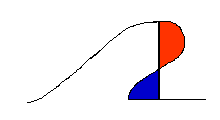The red area is subtracted from the multivalued solution and the blue area is added. The sum of the blue and unshaded areas then makes up the weak solution. In the applet, observe in the shock problems for inviscid Burgers' equation how the plotted weak solutions appear to be applying this equal area rule to the multivalued solutions produced by the method of characteristics.

### Numerical Methods for Conservation Laws

For inviscid Burgers' equation with piecewise constant initial data, we are able to find a formula for the weak solution. For more complicated initial data this will most likely not be the case. Asymptotic formulas (accurate in the limit as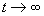, see reference ) for the weak solutions to the problems may exist, but are of little use computationally. Thus, numerical methods that are capable of producing good approximations to the exact entropy satisfying weak solution are important. In general, numerical methods for nonlinear hyperbolic conservation laws are more complicated and difficult to develop than numerical methods for elliptic and parabolic PDEs. For the inviscid Burgers' problem with the sine wave, N-wave, and single hump initial data, the applet uses a numerical method to approximate the exact entropy satisfying weak solution. The method in a second order nonoscillatory finite difference method called Roe's method which employs the used of a flux limiter (the Superbee limiter is used) to suppress numerical oscillations. When using the simulations in the applet that calculate the weak solutions by the numerical method, the ratio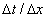must be kept below a certain threshold, or the method will become unstable and the numerical approximation will blow up. The interested reader should consult references  and  for details.

### Inviscid Burgers' equation example problems

The following initial conditions for inviscid Burgers' equation are coded in the applet. The first two problems are Riemann problems, i.e., they have piecewise constant initial data consisting of one discontinuity.

#### Riemann problem - shock

This problem has an initial condition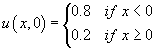. The characteristics intersect and a shock forms immediately for t>0. The exact entropy satisfy weak solution is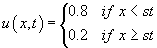where the shock speed is given by. The exact weak solution is plotted in the applet. This problem is discussed in detail in references [1 p. 28], and [3, p. 82].

#### Riemann problem - rarefaction

The initial condition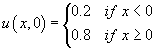produces characteristics (see image below and the applet also) such that there is no characteristic information available in some regions of the x-t plane.A entropy violating weak solution is, where s is the same as the Riemann shock problem above. This solution corresponds to filling in the missing characteristic information as shown in red below.For this problem, it can be shown that infinitely many weak solution exists [1, p. 30].

A entropy satisfying weak solution is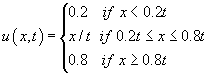. This solution corresponds to filling in the missing characteristic information as shown in red in the image below.This problem is discussed in detail in references [1 p. 28], and [3, p. 82].

#### sine

The initial condition is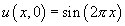. Notice thathas a minimum value of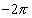. Thus, the breaking time will be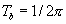(approximately t=0.16) . This problem is discussed in detail in reference [3, p. 77]. The weak solution is computed by using Roe's method.

#### N-wave

The initial condition is. The solution consists of both a left and right moving shock. The weak solution is computed by using Roe's method. This problem is discussed in detail in reference [4, p. 48] and in [1, p. 32]

#### single hump

The initial condition is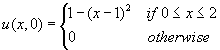. The solutions consist of one right moving shock. The weak solution is computed by using Roe's method. This problem is discussed in detail in reference [4, p. 46]

## Applet

Use of the applet require the Java 1.4 browser plug-in. If you do not have it installed on your computer, the script on the web page should direct you to the site where you can freely get the plug-in and install it. If you do not have the plug-in, and the script does not direct you to the plug-in, follow this link.

The applet provides two views. The window on the left shows the initial condition advancing in time, while the window on the right shows the data advancing along the characteristics in the x-t plane.

Select one of the three example from the equations menu: the advection equation, the variable coefficient advection equation, or inviscid Burgers' equation. The inviscid Burgers' equation option has five submenus corresponding to five different initial conditions. Press the go button to start the animation, the stop button to stop the animation, and the reset button to return to the initial conditions. For inviscid Burgers' equation, the applet can plot the weak solution (as a solid black curve) to the problem. This option may be turned on by checking it on the options menu.

Pressing the parameters button pops up a dialog which allows five parameters to be changed by the user:

dX - Controls the spacing between characteristics on the x axis.
dT- Step size for time advancement. Set dT smaller to slow down the animation or to observe advancement in smaller increments along the characteristics.
final t - The final time at which the animation stops.
speed - Animation speed. The number represents delay in milliseconds. Speed=0 represent no delay and the fastest animation rate.

## References

 Numerical Methods for Conservation Laws. R. LeVeque, Birkhauser, 1992.
 Introduction to Partial Differential Equations with MATLAB. J. Cooper, Birkhauser, 1998.
 Numerical Partial Differential Equations: Conservation Laws and Elliptic Equations. J. Thomas. Springer, 1999.
 Linear and Nonlinear Waves. G.B. Whitham, Wiley, 1974.
 Partial Differential Equations for Scientists and Engineers. Stanley J. Farlow. Dover Publications, 1982.
 Introduction to Applied Mathematics. Gilbert Strang. Wellesley-Cambridge Press, 1986.
 Partial Differential Equations, 4th edition. Fritz John. Springer, 1982.